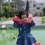# Most Difficult Redox Equation

this is one of the hardest redox equations.

the problem is:

CuSCN + KIO3 + HCl === CuSO4 + KCl + HCN + ICI + H2O

Acid Or Base Does Not Matter.

Please Post Solutions...Note by Anika .
6 years, 9 months ago

This discussion board is a place to discuss our Daily Challenges and the math and science related to those challenges. Explanations are more than just a solution — they should explain the steps and thinking strategies that you used to obtain the solution. Comments should further the discussion of math and science.

When posting on Brilliant:

• Use the emojis to react to an explanation, whether you're congratulating a job well done , or just really confused .
• Ask specific questions about the challenge or the steps in somebody's explanation. Well-posed questions can add a lot to the discussion, but posting "I don't understand!" doesn't help anyone.
• Try to contribute something new to the discussion, whether it is an extension, generalization or other idea related to the challenge.
• Stay on topic — we're all here to learn more about math and science, not to hear about your favorite get-rich-quick scheme or current world events.

MarkdownAppears as
*italics* or _italics_ italics
**bold** or __bold__ bold
- bulleted- list
• bulleted
• list
1. numbered2. list
1. numbered
2. list
Note: you must add a full line of space before and after lists for them to show up correctly
paragraph 1paragraph 2

paragraph 1

paragraph 2

[example link](https://brilliant.org)example link
> This is a quote
This is a quote
    # I indented these lines
# 4 spaces, and now they show
# up as a code block.

print "hello world"
# I indented these lines
# 4 spaces, and now they show
# up as a code block.

print "hello world"
MathAppears as
Remember to wrap math in $$ ... $$ or $ ... $ to ensure proper formatting.
2 \times 3 $2 \times 3$
2^{34} $2^{34}$
a_{i-1} $a_{i-1}$
\frac{2}{3} $\frac{2}{3}$
\sqrt{2} $\sqrt{2}$
\sum_{i=1}^3 $\sum_{i=1}^3$
\sin \theta $\sin \theta$
\boxed{123} $\boxed{123}$

## Comments

Sort by:

Top Newest

Here you go:

$\underline{4}CuSCN+\underline{7}KIO_3+\underline{14}HCl=\underline{4}CuSO_4+\underline{7}KCl+\underline{4}HCN+\underline{7}ICl+\underline{5}H_2O$

- 6 years, 9 months ago

Log in to reply

If you want to solve this problem you have to write out the redox half equations for three reactions:

The oxidation of Copper I to copper II

The oxidation of SCN- to Sulphate

The reduction of iodate V to iodine I (this is the trap as we usually think of Iodine as -1, but in ICl it is behaving like I+)

You need to keep the oxidation equation coefficients equal as Cu=SCN

The common factor for eliminating electrons from the oxidation and reduction equations is 28

Hope this helps...

Periodic Player

- 3 years, 8 months ago

Log in to reply

By redox equations do you mean like $2H_2+O_2=2H_2O$? Ok so I need to balance

$\underline{\hspace{2mm}} CuSCN+\underline{\hspace{2mm}}KIO_3+\underline{\hspace{2mm}}HCl=\underline{\hspace{2mm}}CuSO_4+\underline{\hspace{2mm}}KCl+\underline{\hspace{2mm}}HCN+\underline{\hspace{2mm}}ICl+\underline{\hspace{2mm}}H_2O$

Sounds interesting. I shall give it a try.

P.S. here's the latex for the equation:

\underline{\hspace{2mm}}CuSCN+\underline{\hspace{2mm}}KIO3+\underline{\hspace{2mm}}HCl=\underline{\hspace{2mm}}CuSO4+\underline{\hspace{2mm}}KCl+\underline{\hspace{2mm}}HCN+\underline{\hspace{2mm}}ICl+\underline{\hspace{2mm}}H_2O\

- 6 years, 9 months ago

Log in to reply

If you want to solve this problem you have to write out the redox half equations for three reactions:

The oxidation of Copper I to copper II

The oxidation of SCN- to Sulphate

The reduction of iodate V to iodine I (this is the trap as we usually think of Iodine as -1, but in ICl it is behaving like I+)

You need to keep the two oxidation equation coefficients equal so that n(Cu) = n(SCN)

The common factor for eliminating electrons from the oxidation and reduction equations is 28

Hope this helps...

Periodic Player

- 3 years, 8 months ago

Log in to reply

Plz give the solution to balance the rxn

- 2 years, 5 months ago

Log in to reply

any eqn in chemistry can be balanced by assuming coefficients as 1,a,b,c,d.....simply equate no of each atoms on both sides.

CuSCN + aKIO3 + bHCl === cCuSO4 + dKCl + eHCN + fICI + gH2O

u may get fractions but multiply by lcm of denominators.

But this doesnt mean i can do any Q from chem.... i am very bad at it just like oil with water(does not go together)

- 6 years, 3 months ago

Log in to reply

Are all they in aqeuous state??

- 5 years, 12 months ago

Log in to reply

2CuS- +3IO3- + 2H+======2CuSo4 + 3I- + H2O

- 4 years, 1 month ago

Log in to reply

the question have 2 answer

- 1 year, 4 months ago

Log in to reply

It is hard but I remember having such equation by the last year in the high school

- 8 months, 1 week ago

Log in to reply

Hey there... aCuSCN+bKIO3+cHCl = dCuSO4 +eKCl+fHCN+gICl+ hH2O

I got a=d=f=1 b=7/4 c=7/2 e=b=g=7/4 H=5/4

Multiplying ever coefficient by 4 A=4 B=7 C=14 D=4 E=7 F=4 G=7 H=5 So balanced chemical equation=

4CuSCN+7KIO3+14HCl== 4CuSO4+7KCl+4HCN+7ICl+5H2O

Me of class 10:- Ayush Raj.. Is it right...??

Done by classic algebra

- 2 months, 1 week ago

Log in to reply

1 HCl + KIO3 + CuSCN → H2O + KCl + CuSO4 + HCN + ICl 2 HCl + KIO3 + Cu(SCN) → H2O + KCl + CuSO4 + HCN + ICl

- 2 weeks, 1 day ago

Log in to reply

2CuSCN+3KIO3+6HCl====)2CuSO4+3KCl+2HCN+3ICl+2H2O

- 3 years, 4 months ago

Log in to reply

×

Problem Loading...

Note Loading...

Set Loading...